# Statements

Adaptive Script allows you to execute one or more statements, sequentially. Statements are separated by the `;` character. This programmatic flow lets the programmer write logic procedurally. The following is a list of the statements that are available in Adaptive Script.

## Block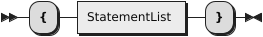A Block is a sequence of statements that are executed sequentially. When creating a script, a new block is created initially. Blocks can be nested inside existing blocks, using braces `{ }`.

### Example

``````{
let x = 1;
let y = 2;
let z = x + y;
print(z);
}``````

## Assignment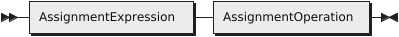The Assignment statement assigns the value of an Expression to an AssignmentExpression. An AssignmentExpression is also an Expression which must evaluate to one of the following:

• VariableReference
• ReferenceByIndex
• ReferenceByName

This flexibility allows us to support a popular technique of assignment known as "destructuring". In destructuring, we assign an object or list into multiple variable names in one assignment.

### Example

``````let x = 1;
let {y} = { y: 2 };
let [z] = [ 3 ];``````

## Break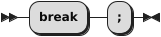The `break` statement breaks out of the current block.

### Example

``````while (true) {
if (1 < 2) {
break;
}
}``````

## Call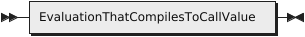A Call statement is an evaluation that compiles to the call of an Adaptive Value.

## Const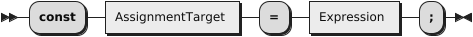The `const` statement declares constant variables.

### Example

``const x = 1;``

## Declare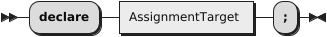## Do While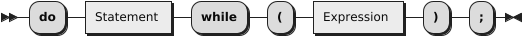The do-while statement executes a block of statements until a condition is met. Unlike the while statement, the do-while statement executes at least once.

### Example

``````let x = 0;
do {
x = x + 1;
} while (x < 10);``````

## For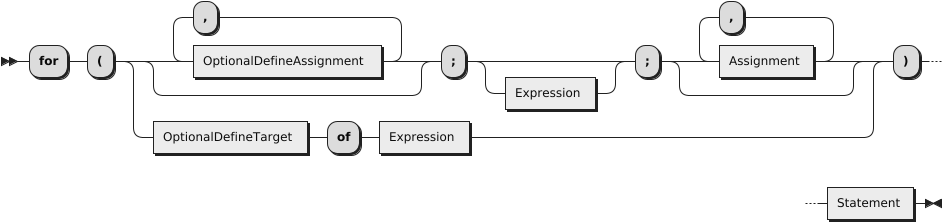The `for` statement executes a block of statements until a condition is met. Unlike the while statement, the for statement executes an initializer, a condition, and an incrementor.

### Example

``````for (let i = 0; i < 10; i = i + 1) {
print(i);
}``````

## Foreach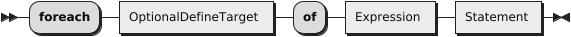### Example

``````foreach let x of [1, 2, 3] {
print(x);
}``````

## Function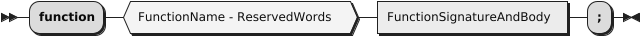This declares a function.

### Example

``````function func(a, b) {
return a + b;
}
function func2(a, b) : a + b;``````

## If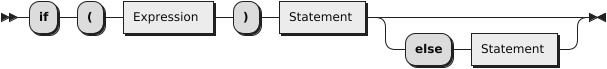The `if` statement executes a block of statements if a condition evaluates to true.

### Example

``````if (1 < 2) {
print(1);
}``````

## Interface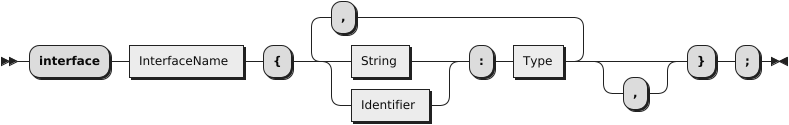## Let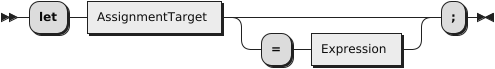The `let` statement declares a local variable.

``let x = 1;``

## Return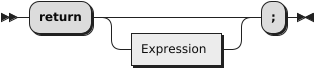The `return` statement returns a value from a block of execution.

### Example

``````function func(a, b) {
return a + b;
}``````

## Switch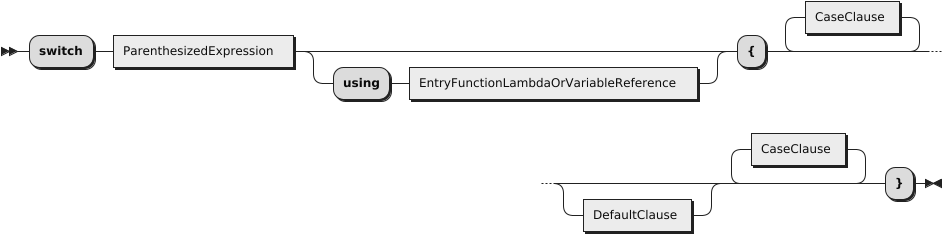The `switch` statement branches control flow based on the value of an expression and the value of each case.

### Example

``````switch (val) {
case 1:
print(1);
break;
case 2:
print(2);
break;
default:
print("default");
break;
}``````

Unlike the `switch` in ECMAScript, Adaptive Script includes an optional syntax that allows you to specify a matching function through the `using` option. The function provided must take two values and return a boolean result. You may use any built-in Adaptive Function, or provide a custom function, written in Adaptive Script.

## Example

``````switch ("abc") using regexp_match {
case "a.*":
// do something
break;
default:
// no match
break;
}``````

## Type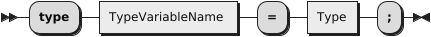## While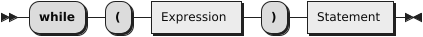The `while` statement executes a block of statements until a condition is met. Unlike the do-while statement, the while statement executes the condition first and may not execute the block at all.

### Example

``````while (false) {
print("This won't execute!");
}``````